# RD Sharma Solutions For Class 12 Maths Exercise 4.11 Chapter 4 Inverse Trigonometric Functions

This exercise explains the topic of properties of inverse trigonometric functions with illustrations. Students can get the detailed RD Sharma Solutions for Class 12 Maths Exercise 4.11 Chapter 4 Inverse Trigonometric Functions from the links available below. Students gain better knowledge about the exercise wise problems in this chapter by solving RD Sharma Solutions for Class 12 as their study material. To score well in the exams, students have to practice the solutions provided by the experts at BYJU’S without fail. Pdfs can be downloaded easily from the links given below.

## Download the PDF of RD Sharma Solutions For Class 12 Chapter 4 – Inverse Trigonometric Functions Exercise 4.11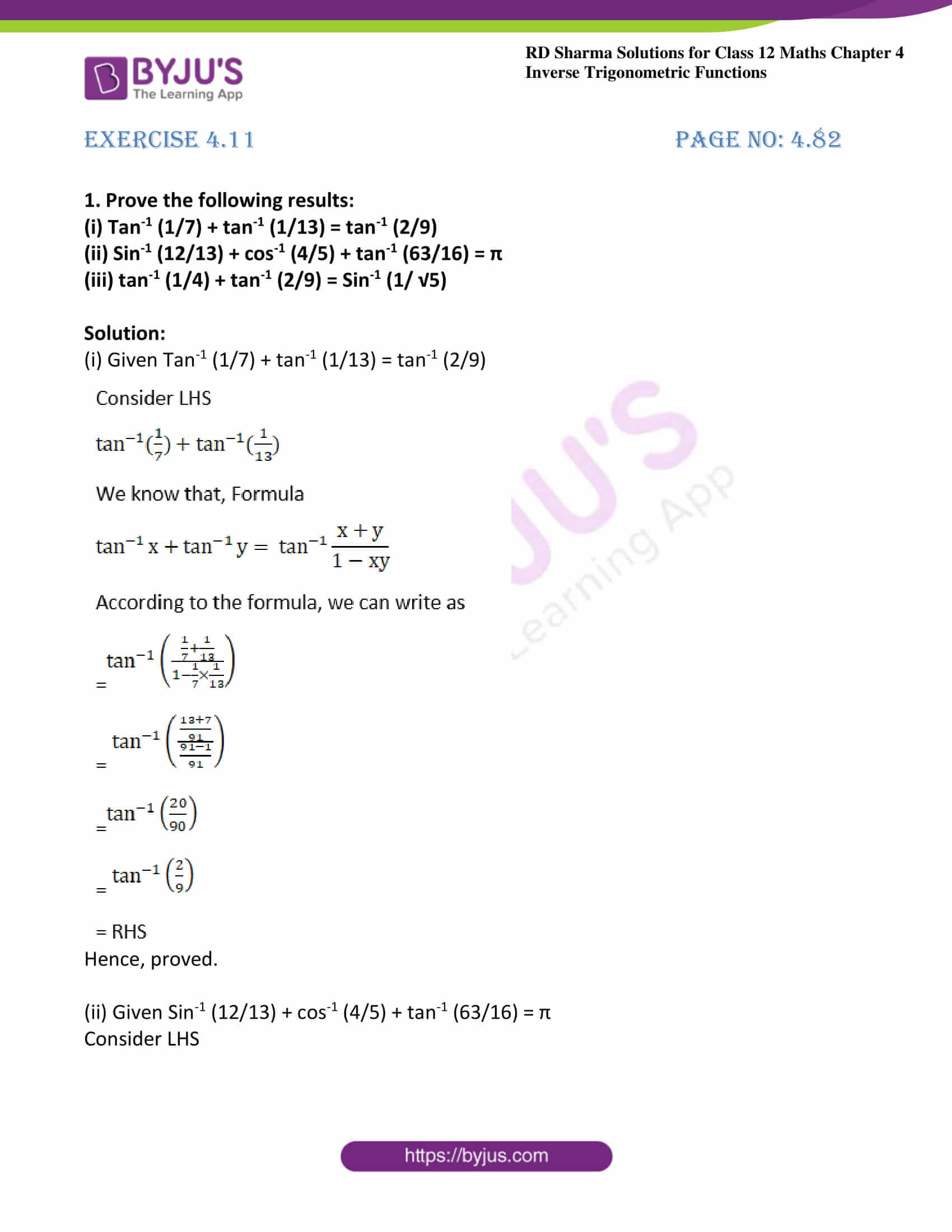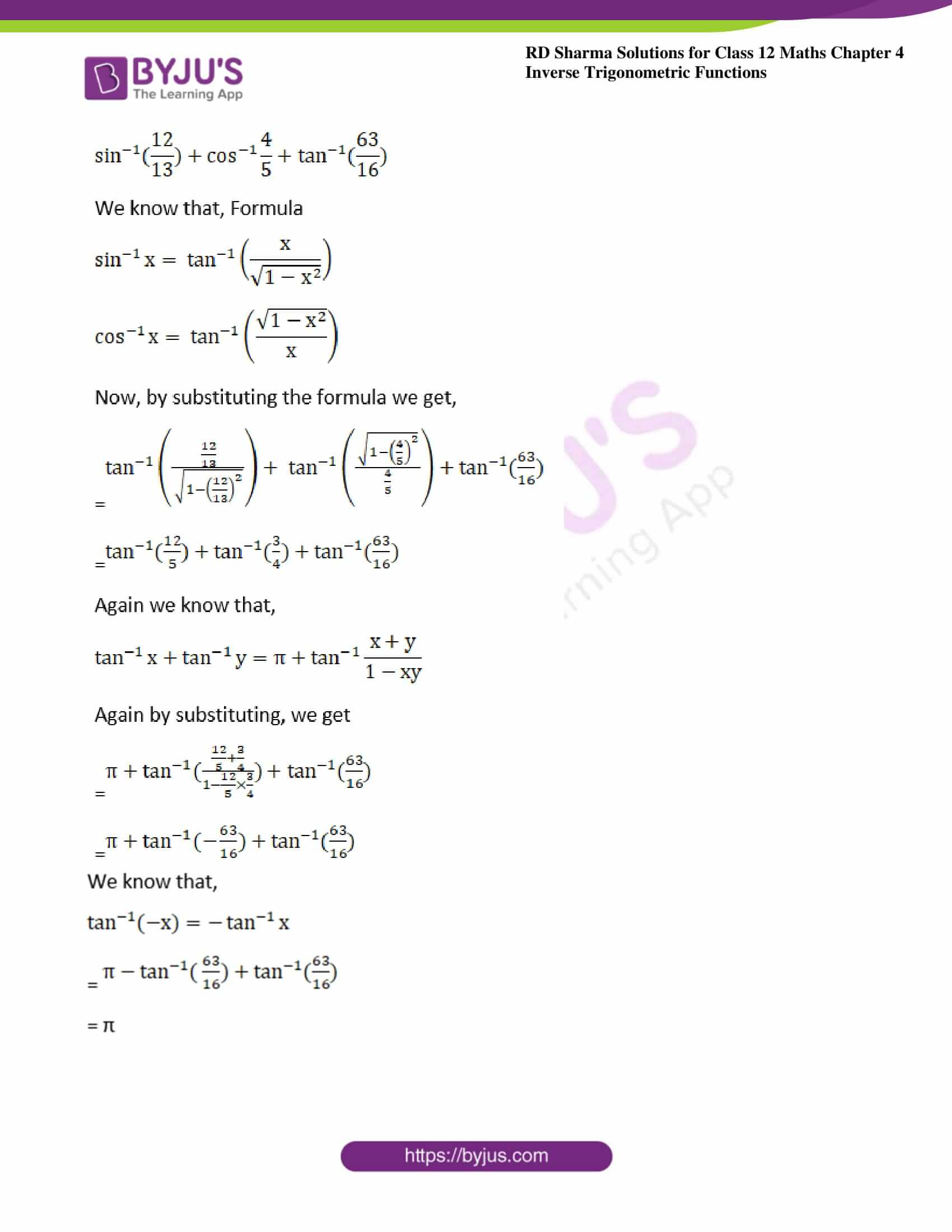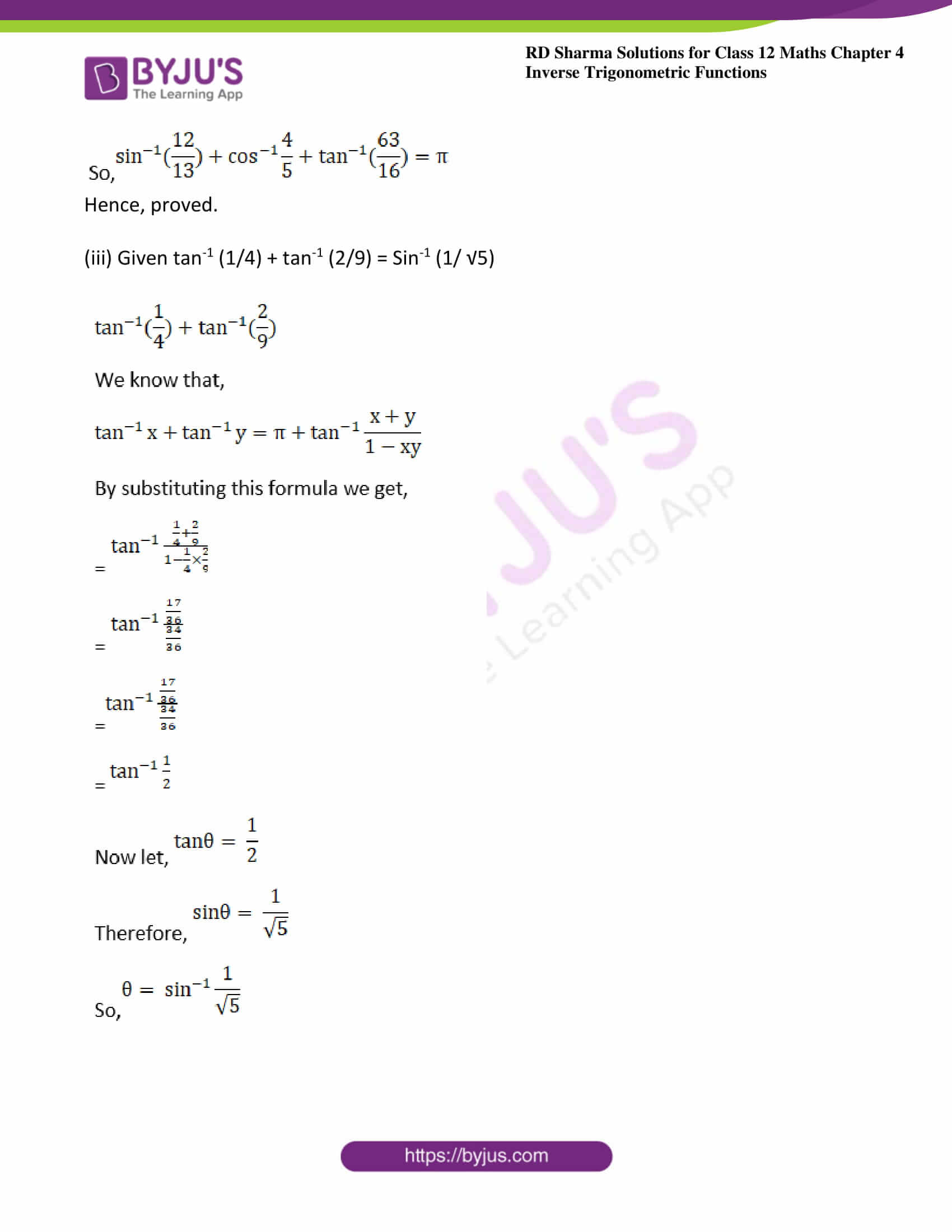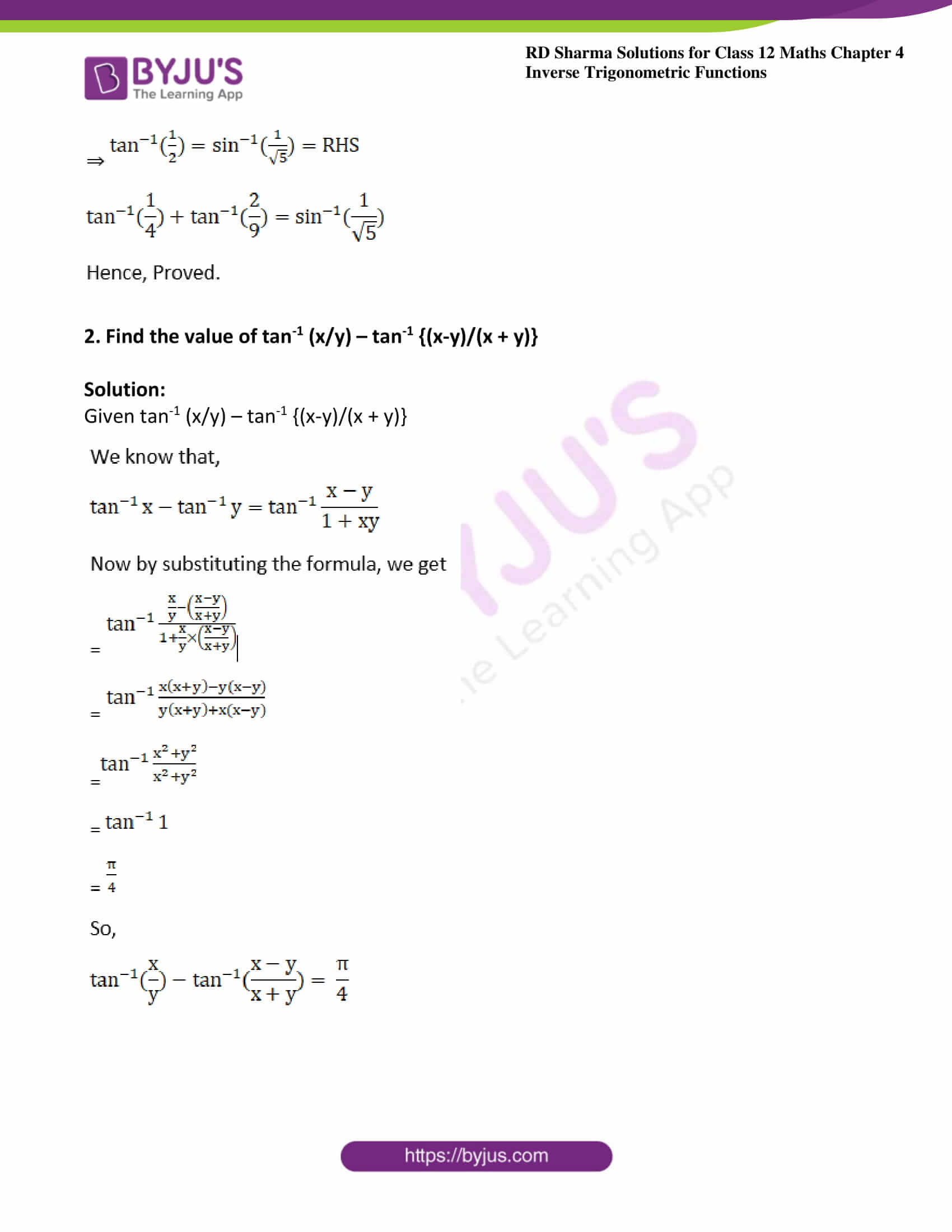### Exercise 4.11 Page No: 4.82

1. Prove the following results:

(i) Tan-1 (1/7) + tan-1 (1/13) = tan-1 (2/9)

(ii) Sin-1 (12/13) + cos-1 (4/5) + tan-1 (63/16) = π

(iii) tan-1 (1/4) + tan-1 (2/9) = Sin-1 (1/ √5)

Solution:

(i) Given Tan-1 (1/7) + tan-1 (1/13) = tan-1 (2/9)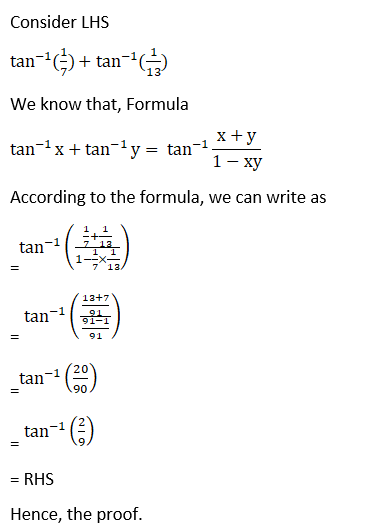Hence, proved.

(ii) Given Sin-1 (12/13) + cos-1 (4/5) + tan-1 (63/16) = π

Consider LHS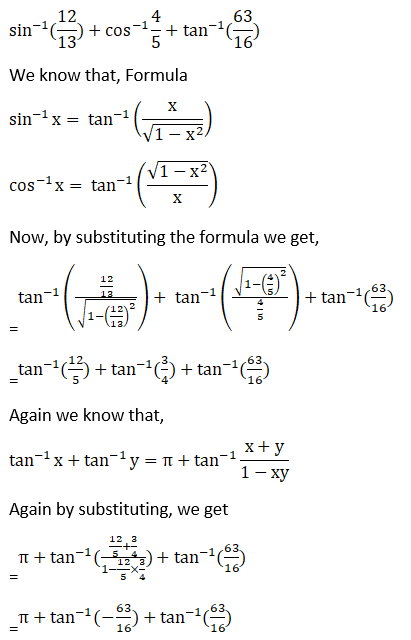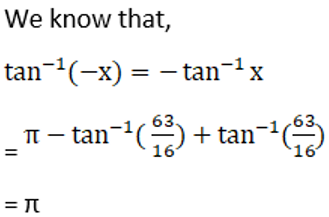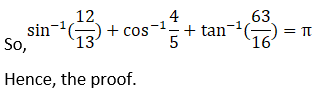Hence, proved.

(iii) Given tan-1 (1/4) + tan-1 (2/9) = Sin-1 (1/ √5)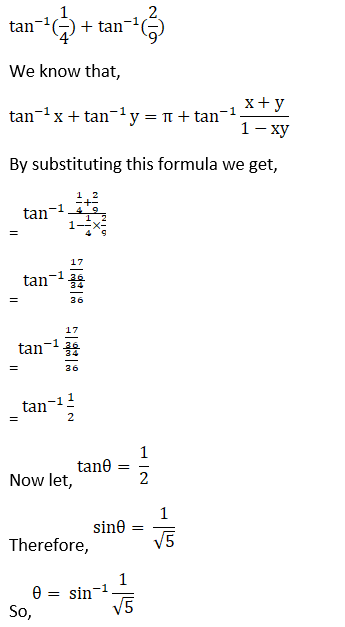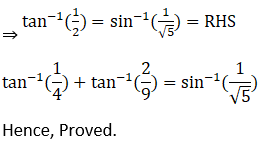2. Find the value of tan-1 (x/y) – tan-1 {(x-y)/(x + y)}

Solution:

Given tan-1 (x/y) – tan-1 {(x-y)/(x + y)}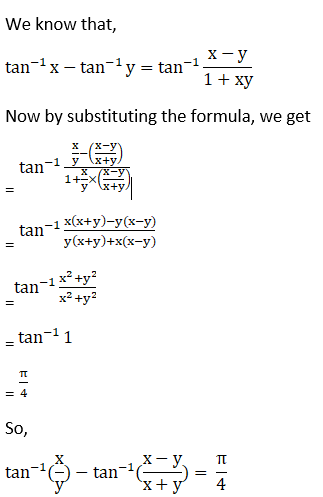### Access other exercises of RD Sharma Solutions For Class 12 Chapter 4 – Inverse Trigonometric Functions

Exercise 4.1 Solutions

Exercise 4.2 Solutions

Exercise 4.3 Solutions

Exercise 4.4 Solutions

Exercise 4.5 Solutions

Exercise 4.6 Solutions

Exercise 4.7 Solutions

Exercise 4.8 Solutions

Exercise 4.9 Solutions

Exercise 4.10 Solutions

Exercise 4.12 Solutions

Exercise 4.13 Solutions

Exercise 4.14 Solutions